# SVM Hyperparameter Tuning using GridSearchCV

March 10th, 2020

This article was written by Clare Liu and originally appeared on the Towards Data Science Blog here: https://towardsdatascience.com/svm-hyper-parameter-tuning-using-gridsearchcv-49c0bc55ce29In my previous article, I have illustrated the concepts and mathematics behind Support Vector Machine (SVM) algorithm, one of the best supervised machine learning algorithms for solving classification or regression problems. It is used in a variety of applications such as face detection, handwriting recognition and classification of emails. In order to show how SVM works in Python including, kernels, hyper-parameter tuning, model building and evaluation on using the Scikit-learn package, I will be using the famous Iris flower dataset to classify the types of Iris flower.

The Iris flower data set is a multivariate data set introduced by Sir Ronald Fisher in the 1936 as an example of discriminant analysis.

The data set consists of 50 samples from each of three species of Iris (Iris setosa, Iris virginica and Iris versicolor), so there are 150 total samples. Four features were measured from each sample: the length and the width of the sepals and petals, in centimetres.

Here’s a picture of the three different Iris species ( Iris setosa, Iris versicolor, Iris virginica). Given the dimensions of the flower, we will predict the class of the flower.

Import the libraries

```import pandas as pd
import numpy as np
from sklearn.svm import SVC
from sklearn.metrics import classification_report, confusion_matrix
import matplotlib.pyplot as plt
%matplotlib inline```

Read the input data from the external CSV

`irisdata = pd.read_csv('iris.csv')`

Take a look at the data

```irisdata.head()
irisdata.info()```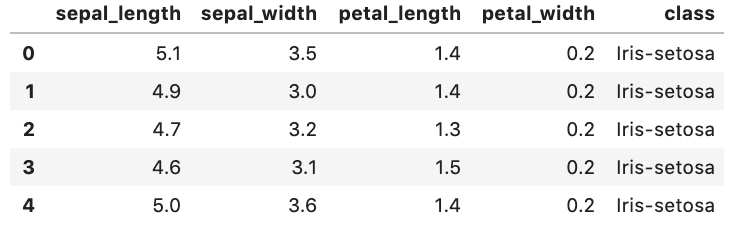The head() function is to return the first 5 rows of the iris data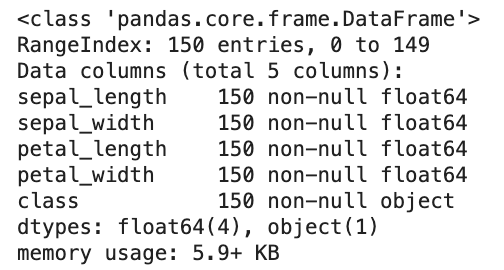info() function is to print a short summary of the iris data

Visualise Data with Pairs Plots

we apply Seaborn which is a library for making statistical graphics in Python. It is built on top of matplotlib and closely integrated with pandas data structures. This function will create a grid of Axes such that each numeric variable in `irisdata` will by shared in the y-axis across a single row and in the x-axis across a single column.

```import seaborn as sns
sns.pairplot(irisdata,hue='class',palette='Dark2')```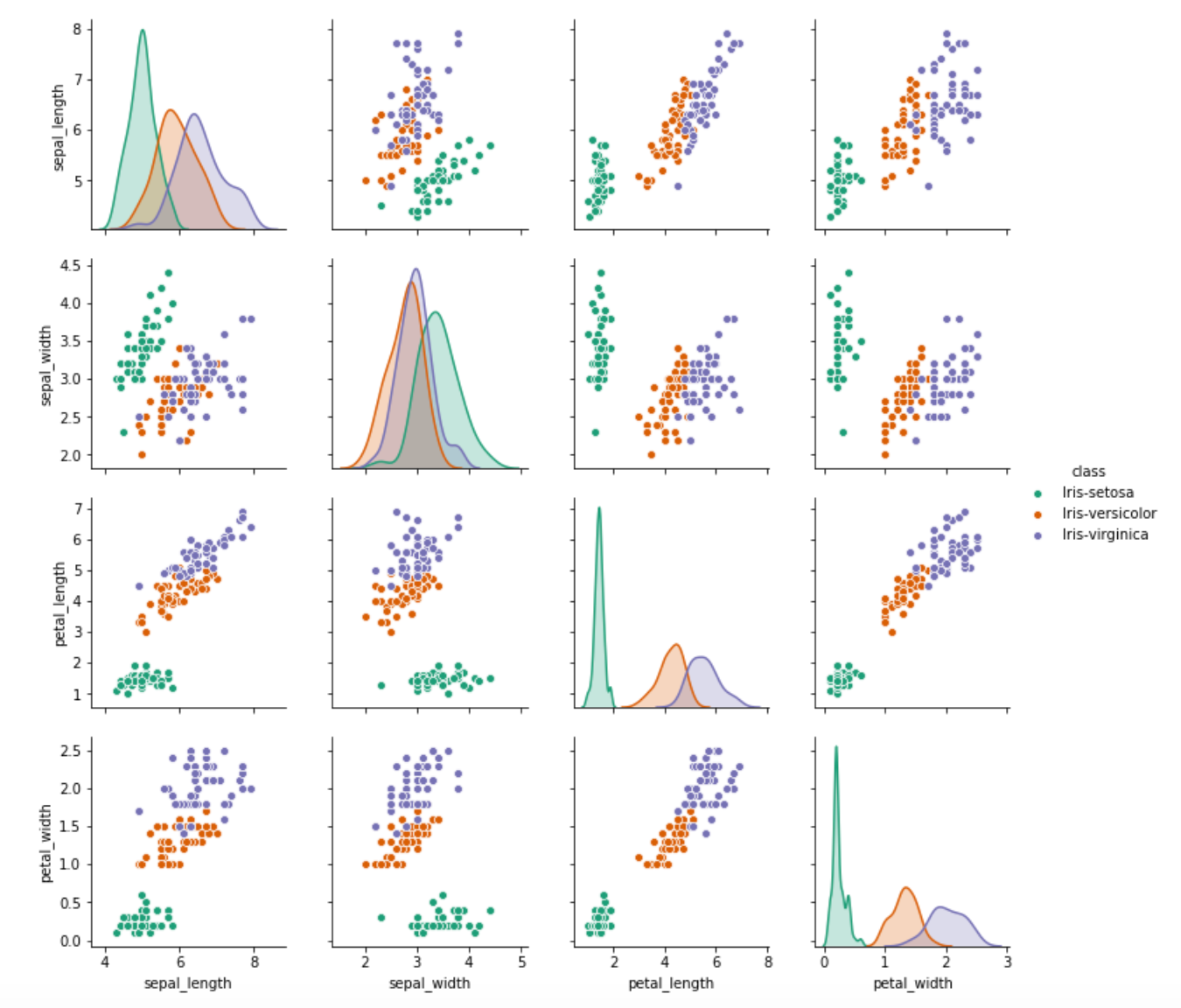A pairs plot allows us to see both distribution of single variables and relationships between two variables.

Train Test Split — Split your data into a training set and a testing set.

```from sklearn.model_selection import train_test_split
X = irisdata.drop('class', axis=1)
y = irisdata['class']
X_train, X_test, y_train, y_test = train_test_split(X, y, test_size = 0.20)```

Apply kernels to transform the data to a higher dimension

```kernels = ['Polynomial', 'RBF', 'Sigmoid','Linear']#A function which returns the corresponding SVC model
def getClassifier(ktype):
if ktype == 0:
# Polynomial kernal
return SVC(kernel='poly', degree=8, gamma="auto")
elif ktype == 1:
return SVC(kernel='rbf', gamma="auto")
elif ktype == 2:
# Sigmoid kernal
return SVC(kernel='sigmoid', gamma="auto")
elif ktype == 3:
# Linear kernal
return SVC(kernel='linear', gamma="auto")```

Train a model

Now it’s time to train a Support Vector Machine Classifier.

Call the SVC() model from sklearn and fit the model to the training data

```for i in range(4):
# Separate data into test and training sets
X_train, X_test, y_train, y_test = train_test_split(X, y, test_size = 0.20)# Train a SVC model using different kernal
svclassifier = getClassifier(i)
svclassifier.fit(X_train, y_train)# Make prediction
y_pred = svclassifier.predict(X_test)# Evaluate our model
print("Evaluation:", kernals[i], "kernel")
print(classification_report(y_test,y_pred))```

Since SVMs is suitable for small data set: `irisdata`, the SVM model would be good with high accuracy expect using Sigmoid kernels. We could be able to determine which kernel performs the best based on the performance metrics such as precision, recall and f1 score.

In order to improve the model accuracy, there are several parameters need to be tuned. Three major parameters including:

1. Kernels: The main function of the kernel is to take low dimensional input space and transform it into a higher-dimensional space. It is mostly useful in non-linear separation problem.

2. C (Regularisation): C is the penalty parameter, which represents misclassification or error term. The misclassification or error term tells the SVM optimisation how much error is bearable. This is how you can control the trade-off between decision boundary and misclassification term.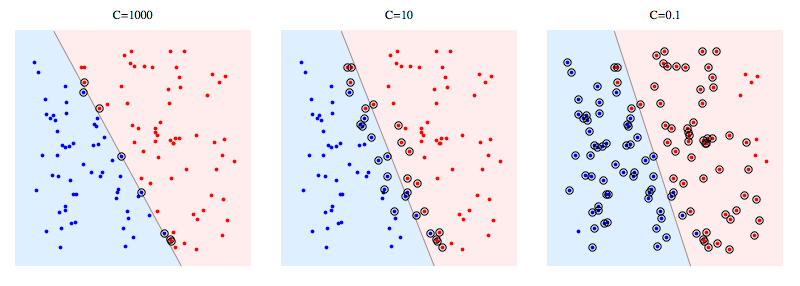when C is high it will classify all the data points correctly, also there is a chance to overfit.

3. Gamma: It defines how far influences the calculation of plausible line of separation.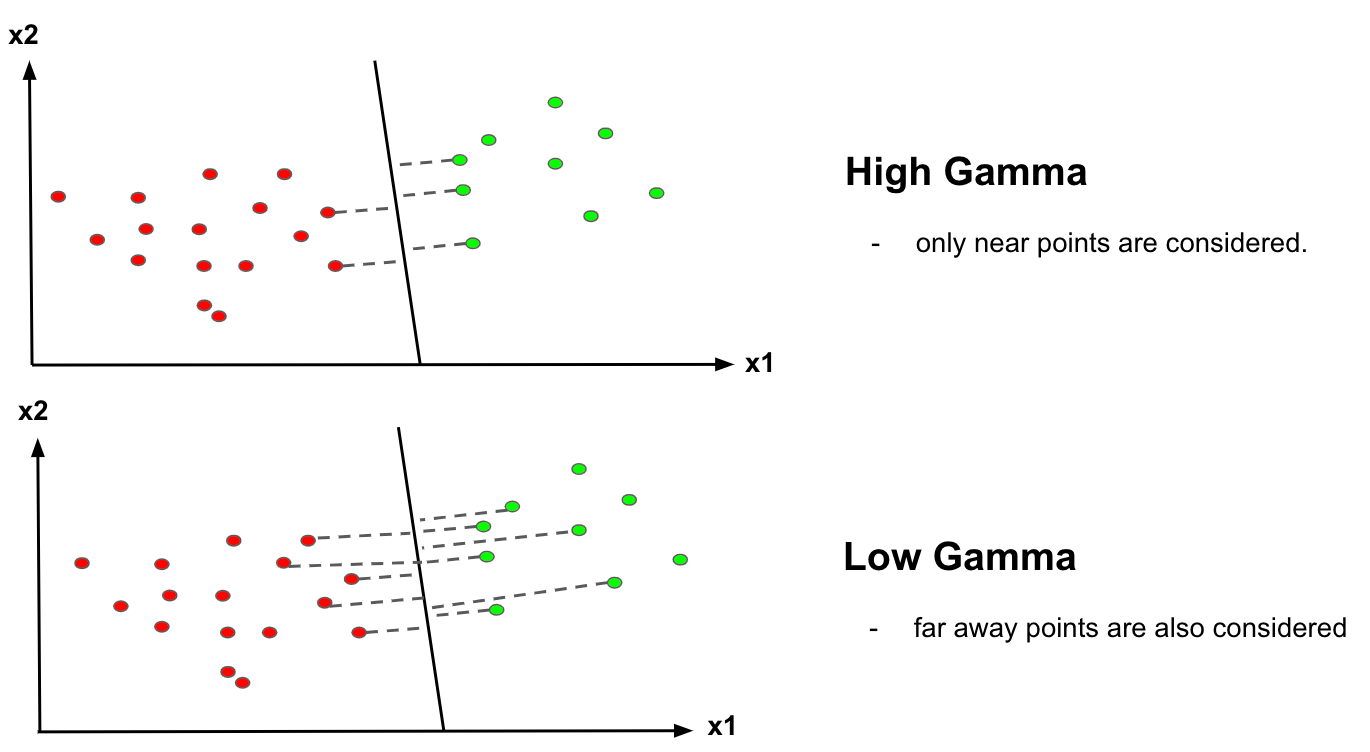when gamma is higher, nearby points will have high influence; low gamma means far away points also be considered to get the decision boundary.

Hyper-parameters are parameters that are not directly learnt within estimators. In scikit-learn, they are passed as arguments to the constructor of the estimator classes. Grid search is commonly used as an approach to hyper-parameter tuning that will methodically build and evaluate a model for each combination of algorithm parameters specified in a grid.

GridSearchCV helps us combine an estimator with a grid search preamble to tune hyper-parameters.

Import GridsearchCV from Scikit Learn

`from sklearn.model_selection import GridSearchCV`

Create a dictionary called param_grid and fill out some parameters for kernels, C and gamma

`param_grid = {'C': [0.1,1, 10, 100], 'gamma': [1,0.1,0.01,0.001],'kernel': ['rbf', 'poly', 'sigmoid']}`

Create a GridSearchCV object and fit it to the training data

```grid = GridSearchCV(SVC(),param_grid,refit=True,verbose=2)
grid.fit(X_train,y_train)```

Find the optimal parameters

`print(grid.best_estimator_)`

Take this grid model to create some predictions using the test set and then create classification reports and confusion matrices

```grid_predictions = grid.predict(X_test)
print(confusion_matrix(y_test,grid_predictions))
print(classification_report(y_test,grid_predictions))#Output
[[15  0  0]
[ 0 13  1]
[ 0  0 16]]```
• Visualise data with Pairs Plots
• Understand three major parameters of SVMs: Gamma, Kernels and C (Regularisation)
• Apply kernels to transform the data including ‘Polynomial’, ‘RBF’, ‘Sigmoid’, ‘Linear’
• Use GridSearch to tune the hyper-parameters of an estimator

Thank you for reading. Hope you now understand how to build the SVMs in Python. Please leave your comments below if you have any thoughts.